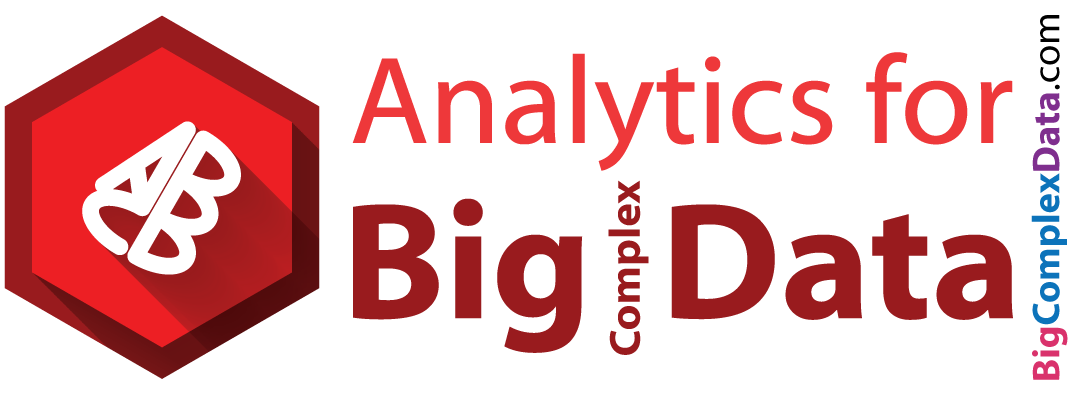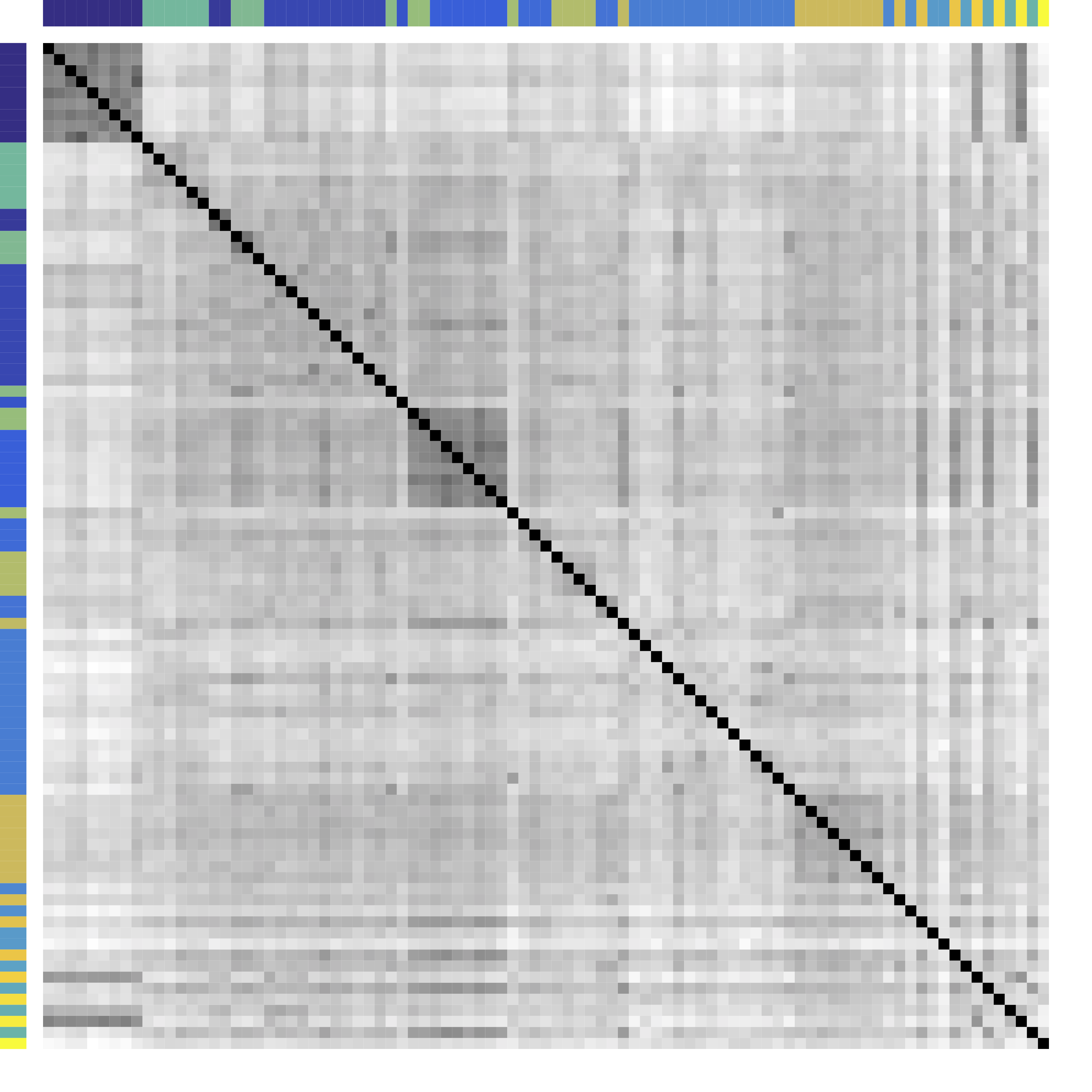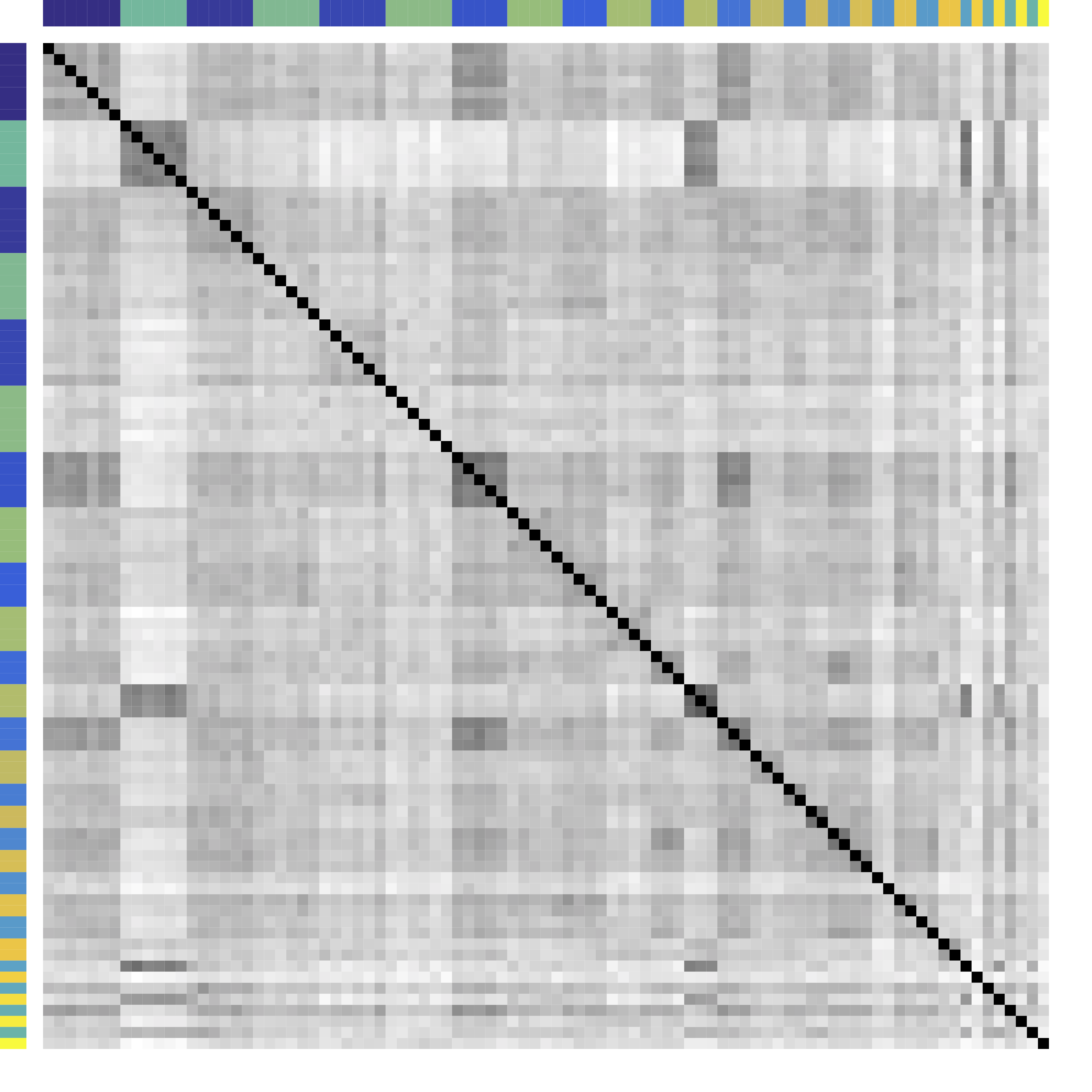$$\def\RR{\bf R} \def\real{\mathbb{R}} \def\bold#1{\bf #1} \def\d{\mbox{Cord}} \def\hd{\widehat \mbox{Cord}} \DeclareMathOperator{\cov}{cov} \DeclareMathOperator{\var}{var} \DeclareMathOperator{\cor}{cor} \newcommand{\ac}{\left\{#1\right\}} \DeclareMathOperator{\Ex}{\mathbb{E}} \DeclareMathOperator{\diag}{diag}$$

## Variable Clustering via $G$-Models of Large Covariance Matrices

### Xi (Rossi) Luo

Brown University
Department of Biostatistics
Center for Statistical Sciences
Computation in Brain and Mind
Brown Institute for Brain Science
ABCD Research GroupICSA 2016, Shanghai, CHINA
December 20, 2016

Funding: NIH R01EB022911; NSF/DMS (BD2K) 1557467; NIH P20GM103645, P01AA019072, P30AI042853; AHA

## CollaboratorsFlorentina Bunea
Cornell UniversityChristophe Giraud
Paris Sud University

## Big Data Problem

• We are interested in big cov with many variables
• Global property for certain joint distributions
• Real-world cov: maybe non-sparse and other structures
• Clustering successful for Big Data Science Donoho, 2015
• Exploratory Data Analysis (EDA)Tukey, 1977
• Hierarchical clustering and KmeansHartigan & Wong, 1979
• Mostly based on marginal/pairwise distances
• Can we combine clustering and big cov estimation?

## Example: SP 100 Data

• Daily returns from stocks in SP 100
• Stocks listed in Standard & Poor 100 Indexas of March 21, 2014
• between January 1, 2006 to December 31, 2008
• Each stock is a variable
• Cov/Cor matrices (Pearson's or Kendall's tau)
• Re-order stocks by clusters
• Compare cov patterns with different clustering/ordering

## Cor after Grouping by ClustersKmeansOur $G$-models

Ours yields stronger off-diagonal, tile patterns. Black = 1.
Color bars: variable groups/clusters
Off-diagonal: correlations across clusters

## Clustering Results

Industry Ours Kmeans Hierarchical Clustering
Home Improvement Home Depot, Lowe’s Home Depot, Lowe’s, Starbucks, Target Home Depot, Lowe’s, Starbucks, Target, Costco, Target, Wal-Mart, FedEx, United Parcel Service, Nike, McDonald’s
Telecom ATT, Verizon ATT, Verizon, Exelon, Comcast, Walt Disney, Time Warner ATT, Verizon, Comcast, Walt Disney, Time Warner, AIG, Allstate, Metlife, American Express, Bank of America, Citigroup, US Bancorp, Wells Fargo, Capital One, Goldman Sachs, JP Morgan Chase, Morgan Stanley, Simon Property, General Electric
Diversified Metals & Mining Freeport-McMoran Freeport-McMoran, National Oillwell Varco Freeport-McMoran, Apache Corp., Anadarko Petroleum, Devon Energy, Halliburton, National Oillwell Varco, Occidental Petroleum, Schlumberger, ConocoPhillips, Chevron, Exxon
$\cdots$
All methods yield 20 clusters.

# Model

## Problem

• Let ${X} \in \real^p$ be a zero mean random vector
• In certain problems, means are arbitrary
• Divide variables into partitions/clusters
• Example: $\{ \{X_1, X_3, X_7\}, \{X_2, X_5\}, \dotsc \}$
• Theoretical: Find a partition $G = \{G_k\}_{ 1 \leq k \leq K}$ of $\{1, \ldots, p\}$ such that all $X_a$ with $a \in G_k$ are "similar"
• Big Data: "helpful" clustering that shows patterns

## Related Areas

• Clustering: Kmeans and Hierarchical Clustering
• Usually for clustering $n$ observations in $R^p$
• Limitations: low signal-noise-ratio, theory, NP-hard
• Q: How to choose number of clusters? Theory?
• Q: Can clusters contain singletons?
• Community detection: huge literature see review Newman, 2003 but start with observed adjacency matrices or networks
• Ours for data that can be generated from unknown networks
• These are related but different problems

## Model: Starting Point

$$X_{n\times p}=\underbrace{Z_{n\times k}}_\text{Source/Factor} \quad \underbrace{G_{k\times p}}_\text{Mixing/Loading} + \underbrace{E_{n\times p}}_{Error} \qquad Z \bot E$$

• Clustering: $G$ is $0/1$ matrix for $k$ clusters/ROIs
• Decomposition:
• PCA/factor analysis: orthogonality
• ICA: orthogonality → independence
• matrix decomposition: e.g. non-negativity
• This model leads to block patterns in $\cov(X)$
• $\cov(X) = G^T \cov(Z) G + \cov(E)$
• Note: not necessarily block-diagonal

## Generalization: $G$-Block

• Example: $G=\ac{\ac{1,2};\ac{3,4,5}}$, $X \in \real^p$ is $G$-block
$$\Sigma =\left(\begin{array}{ccccc} {\color{red} D_1} & {\color{red} C_{11} }&C_{12} & C_{12}& C_{12}\\ {\color{red} C_{11} }&{\color{red} D_1 }& C_{12} & C_{12}& C_{12} \\ C_{12} & C_{12} &{\color{green} D_{2}} & {\color{green} C_{22}}& {\color{green} C_{22}}\\ C_{12} & C_{12} &{\color{green} C_{22}} &{\color{green} D_2}&{\color{green} C_{22}}\\ C_{12} & C_{12} &{\color{green} C_{22}} &{\color{green} C_{22}}&{\color{green} D_2} \end{array}\right) \qquad C = \left(\begin{array}{cc} {\color{red} C_{11} } & C_{12}\\ C_{12} & {\color{green} C_{22}} \end{array}\right)$$
• Matrix math: $\cov(X) = \Sigma = G^TCG + d$
• We allow $|C_{11} | \lt | C_{12} |$ or $C \prec 0$
• Kmeans/HC leads to block-diagonal cor matrices (permutation)
• Clustering based on $G$-Block
• From $G$-block we can read out "negative" $\cov(Z)$
• Cov defined for semiparametric distributions
• Clusters can contain singletons

## Minimum $G$ Partition

Theorem: $G^{\beta}(X)$ is the minimal partition induced by $a\stackrel{G^{\beta}}{\sim} b$
iff $\var(X_{a})=\var(X_{b})$ and $\cov(X_{a},X_{c})=\cov(X_{b},X_{c})$ for all $c\neq a,b$. Moreover, if the matrix of covariances $C$ corresponding to the partition $G(X)$ is positive-semidefinite, then this is the unique minimal partition according to which ${X}$ admits a latent decomposition.
• We define the minimal cluster/partition.
• The minimal partition is unique under conditions.
• We will aim to recover the minimal partition (thus $K$).

# Method

## New Metric: CORD

• First, pairwise correlation distance (like Kmeans)
• Gaussian copula: $$Y:=(h_1(X_1),\dotsc,h_p(X_p)) \sim N(0,R)$$
• Let $R$ be the correlation matrix
• Gaussian: Pearson's
• Gaussian copula: Kendall's tau transformed, $R_{ab} = \sin (\frac{\pi}{2}\tau_{ab})$
• Second, introduce CORrelation Distance $$\d(a,b) := \max_{c\neq a,b}|R_{ac}-R_{bc}|$$
• Third, group variables $a$, $b$ together if $\d(a,b) = 0$
• Do not care any pairwise distance between $a,b$
• "The enemy of my enemy is my friend"

## Algorithm: Main Idea

• Greedy: one cluster at a time, avoiding NP-hard
• Cluster variables together if CORD metric $$\widehat \d(a,b) \lt \alpha$$ where $\alpha$ is a tuning parameter
• $\alpha$ is chosen by theory or CV

# Theory

## Condition

Let $\eta \geq 0$ be given. Let ${ X}$ be a zero mean random vector with a Gaussian copula distribution with parameter $R$. $$\begin{multline} \mathcal{R}(\eta) := \{R: \ \d(a,b) := \max_{c\neq a,b}|R_{ac}-R_{bc}|>\eta\quad \\ \textrm{for all}\ a\stackrel{G(X)}{\nsim}b.\} \end{multline}$$ Group separation condition: $R \in \mathcal{R}(\eta)$.

The signal strength $\eta$ is large.

## Consistency

Theorem: Define $\tau=|\widehat R-R|_{\infty}$ and we consider two parameters $(\alpha,\eta)$ fulfilling $$\begin{equation} \alpha\geq 2\tau\quad\textrm{and}\quad \eta\geq2\tau+\alpha. \end{equation}$$ Then, applying our algorithm we have $\widehat G=G(X)$ whp.

Ours recovers the exact clustering with high probability.

## Minimax

Theorem: $P_{\Sigma}$ the likelihood based on $n$ independent observations of ${ X} \stackrel{d}{=} \mathcal{N}(0,\Sigma)$. For any \begin{equation} 0\leq \eta < \eta^{*}:=\frac{0.6\sqrt{\frac{ \log(p)}{n}}}{1+0.6\sqrt{\frac{ \log(p)}{n}}} \end{equation} we have $$\inf_{\widehat G}\sup_{R \in \mathcal{R}(\eta)} P_{\Sigma}(\widehat G\neq G^{\beta}(X))\geq {1\over 2e+1}\geq {1\over 7} \,,$$ where the infimum is taken over all possible estimators.

Group separation condition on $\eta$ is optimal.

## Choosing Number of Clusters

• Split data into 3 parts
• Use part 1 of data to estimate clusters $\hat{G}$ for each $\alpha$
• Use part 2 to compute between variable difference $$\delta^{(2)}_{ab} = R_{ac}^{(2)} - R_{bc}^{(2)}, \quad c \ne a, b.$$
• Use part 3 to generate "CV" loss $$\mbox{CV}(\hat{G}) = \sum_{a \lt b} \| \delta^{(3)}_{ab} - \delta^{(2)}_{ab} 1\{ a \mbox{ not clustered w/ } b \} \|^2_\infty.$$
• Pick $\alpha$ with the smallest loss above

## Theory for CV

Theorem: If either: (i) $X$ is sub-Gaussian with correlation matrix $R$; or (ii) $X$ has a copula distribution with copula correlation matrix $R$, then we have $E[\mbox{CV}(G^*)] \lt E[\mbox{CV}(G)]$, for any $G\ne G^*$.
This shows that our CV will select $G^*$ consistently.

# Simulations

## Setup

• Model $C$ ($\cov(Z)$): positive semidefinite or negative
• True $G^*$: singletons or no-singleton clusters
• Simulate $X$ from $G$-block cov
• Variable clustering using $X$
• Compare with K-means or Hierarchical Clustering:
• Exact recovery of groups
• Cross validation loss and choosing $K$

## Exact Recovery

Different models for $C$="$\cov(Z)$" and $G$

HC and Kmeans fail even if inputting the true $K$ and $n \rightarrow \infty$

Our CORD methods recover both the true $G^*$ and $K$ as predicted by our theory.

## Cross Validation

Recovery % in red and CV loss in black.

CV selects the constants to yield close to 100% recovery, as predicted by our theory (at least for large $n>200$)

# Real Data

## Functional MRI

• fMRI matrix: BOLD from different brain regions
• Variable: different brain regions
• Sample: time series (after whitening or removing temporal correlations)
• Clusters of brain regions
• Two data matrices from two scan sessions OpenfMRI.org
• Use Power's 264 regions/nodes

## Test Prediction/Reproducibilty

• Find partitions using the first session data
• Average each block cor to improve estimation
• Compare with the cor matrix from the second scan $$\| Avg_{\hat{G}}(\hat{\Sigma}_1) - \hat{\Sigma}_2 \|$$
• Difference is smaller if clustering $\hat{G}$ is better

Vertical lines: fixed (solid) and data-driven (dashed) thresholds

Our CORD $\hat{G}$ leads to smaller between-session variability for almost all $K$, than HC and Kmeans.

## Discussion

• Cov + clustering:
• Identifiability, accuracy, optimality
• $G$-models: $G$-latent, $G$-block, $G$-exchangeable
• New metric, method, and theory
• Defining clusters, consistency, minimax, and CV theory
• Some new results using big data examples
• Paper: bit.ly/cordCluster (arXiv 1508.01939)
• R package: cord on CRAN
• CV function available soon

# Thank you!

## Slides at: bit.ly/ICSA2016## Website: BigComplexData.com

Postdoc position available
funded by Whitehouse's Big Data and BRAIN Initiatives